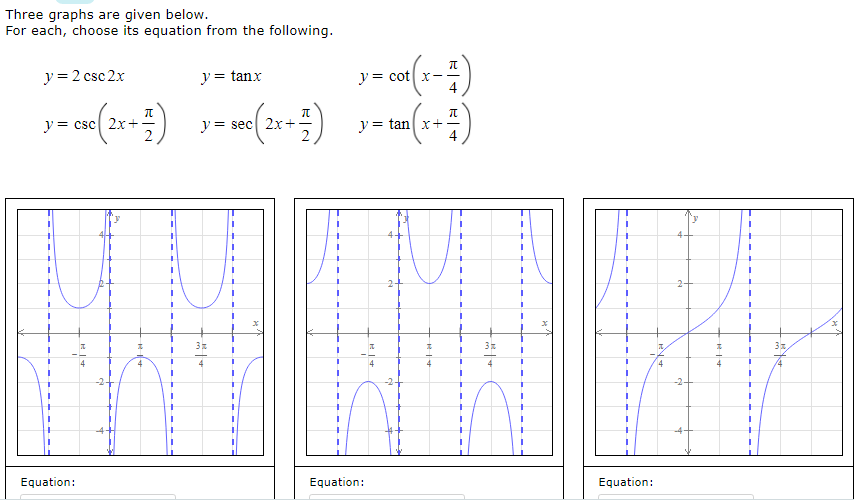# Three graphs are given below. For each, choose its equation from the following. y = 2 csc 2x y = tanx y = cot x- y= csc 2x+ y= sec 2x+. y = tan x+- 2- %3D 4 %3D -2+ -2- 1.

Question

Which of the functions match the three graphs?help_outlineImage TranscriptioncloseThree graphs are given below. For each, choose its equation from the following. y = 2 csc 2x y = tanx y = cot x- y= csc 2x+ y= sec 2x+. y = tan x+- 2- %3D 4 %3D -2+ -2- 1. fullscreen

### Want to see this answer and more?

Experts are waiting 24/7 to provide step-by-step solutions in as fast as 30 minutes!*

*Response times may vary by subject and question complexity. Median response time is 34 minutes for paid subscribers and may be longer for promotional offers.
Tagged in
Math
Algebra

### Other